Authors: William Gearty & Lewis A. Jones

Last updated: 2023-10-28

# Introduction

Herein we provide three example applications of the rphylopic package in combination with the {ggplot2} package. However, note that all demonstrated functionality is also available for base R and showcased in a separate vignette.

# Basic accession and transformation

The rphylopic package provides robust and flexible tools to access and transform PhyloPic silhouettes. Here we demonstrate this using the example dataset of Antarctic penguins from the {palmerpenguins} R package.

First, let’s load our libraries and the penguin data:

# Load libraries
library(rphylopic)
library(ggplot2)
library(palmerpenguins)
# Get penguin data and clean it
data(penguins)
penguins_subset <- subset(penguins, !is.na(sex))

Now, let’s pick a silhouette to use for the penguins. Let’s pick #2:

# Pick a silhouette for Pygoscelis (here we pick #2)
penguin <- pick_phylopic("Pygoscelis", n = 3, view = 3)

You may have noticed in the preview that the silhouette was a little slanted. Let’s rotate it clockwise just a smidgen:

# It's a little slanted, so let's rotate it a little bit
penguin_rot <- rotate_phylopic(img = penguin, angle = 15)

Now, let’s draft the plot that we want to make. In this case, let’s plot the penguins’ bill lengths vs. their flipper lengths:

ggplot(penguins_subset) +
geom_point(aes(x = bill_length_mm, y = flipper_length_mm)) +
labs(x = "Bill length (mm)", y = "Flipper length (mm)") +
facet_wrap(~species, ncol = 1) +
theme_bw(base_size = 15)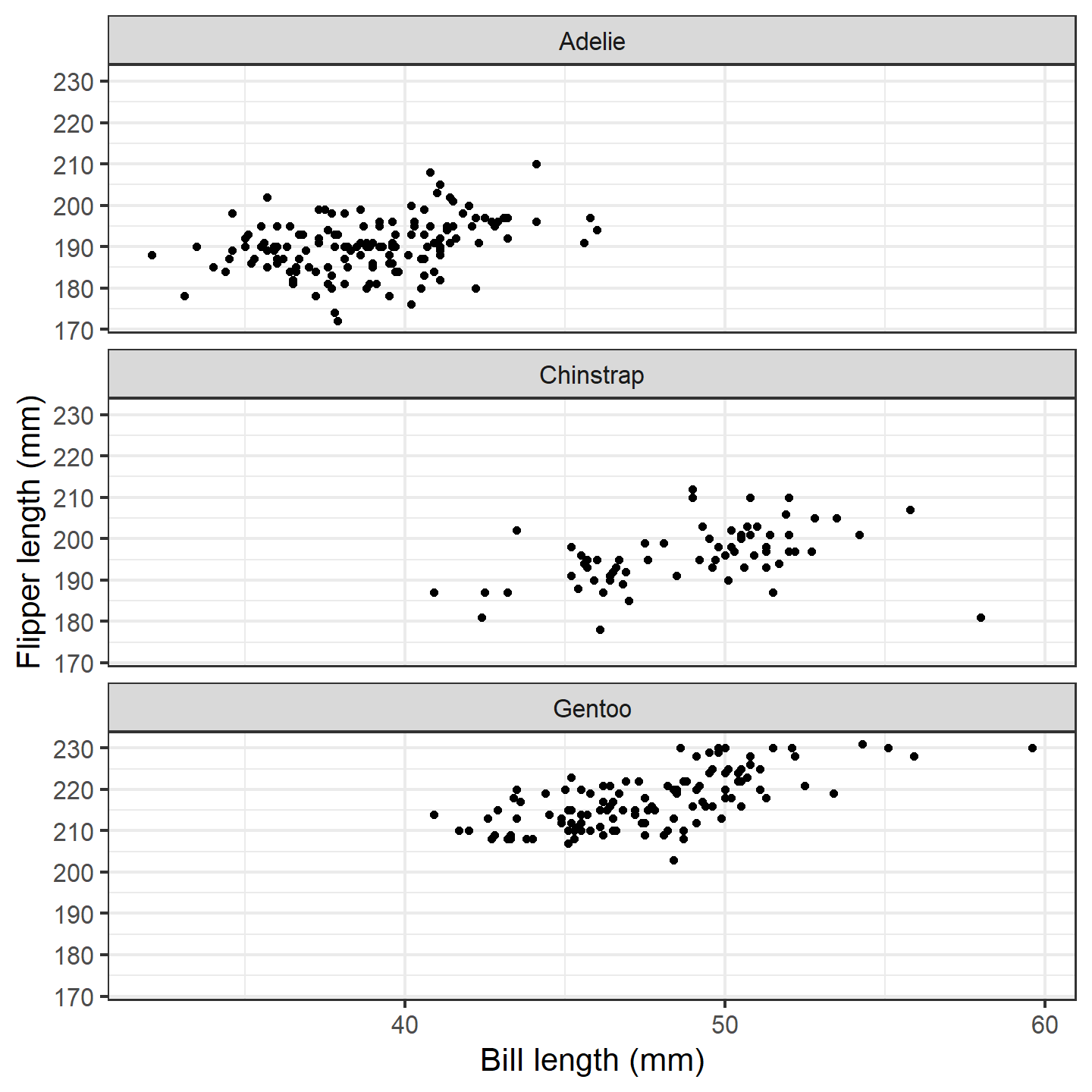plot of chunk ggplot-penguin-plot-1

That’s a nice basic plot! But you know what would make it nicer? If we added a penguin silhouette to the plot. Sadly, we don’t have a different silhouette for each species (although we could make one…), so let’s just go with putting a single silhouette in the top panel. We’ll use the geom_phylopic() function, which will require us to make a data.frame. Note that the x and y aesthetics specify the center of the silhouette, and the size argument specifies how tall the silhouette is in the units of the y-axis.

silhouette_df <- data.frame(x = 59, y = 215, species = "Adelie")
ggplot(penguins_subset) +
geom_point(aes(x = bill_length_mm, y = flipper_length_mm)) +
geom_phylopic(data = silhouette_df, aes(x = x, y = y), size = 30,
img = penguin_rot) +
labs(x = "Bill length (mm)", y = "Flipper length (mm)") +
facet_wrap(~species, ncol = 1) +
theme_bw(base_size = 15)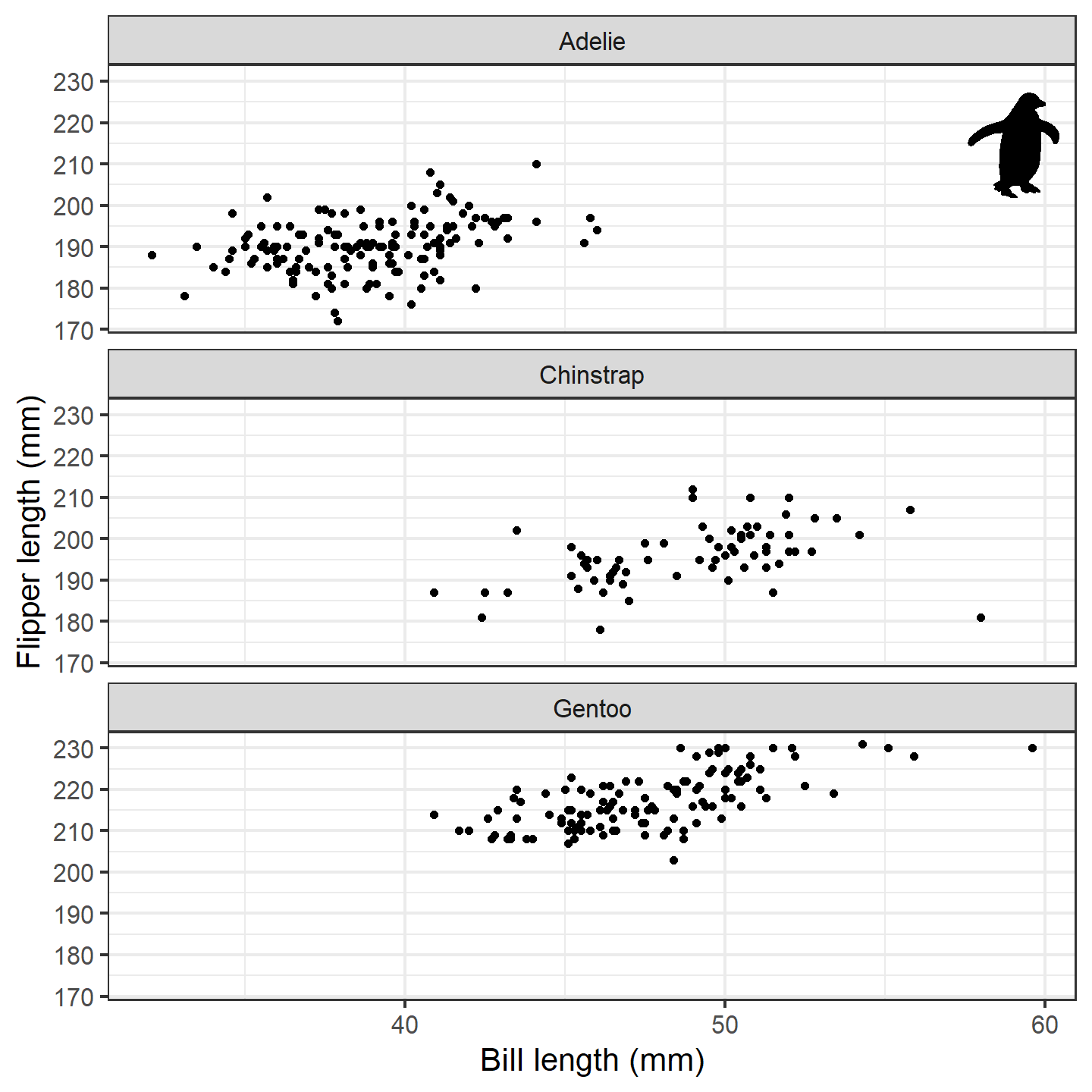plot of chunk ggplot-penguin-plot-2

Isn’t that nifty! We can go a step further, though. What if we used little penguins instead of points?! To do that, we can use the geom_phylopic() function instead of the geom_point() function (in this case, we want to use the same image for each x-y pair):

ggplot(penguins_subset) +
geom_phylopic(img = penguin_rot,
aes(x = bill_length_mm, y = flipper_length_mm)) +
labs(x = "Bill length (mm)", y = "Flipper length (mm)") +
facet_wrap(~species, ncol = 1) +
theme_bw(base_size = 15)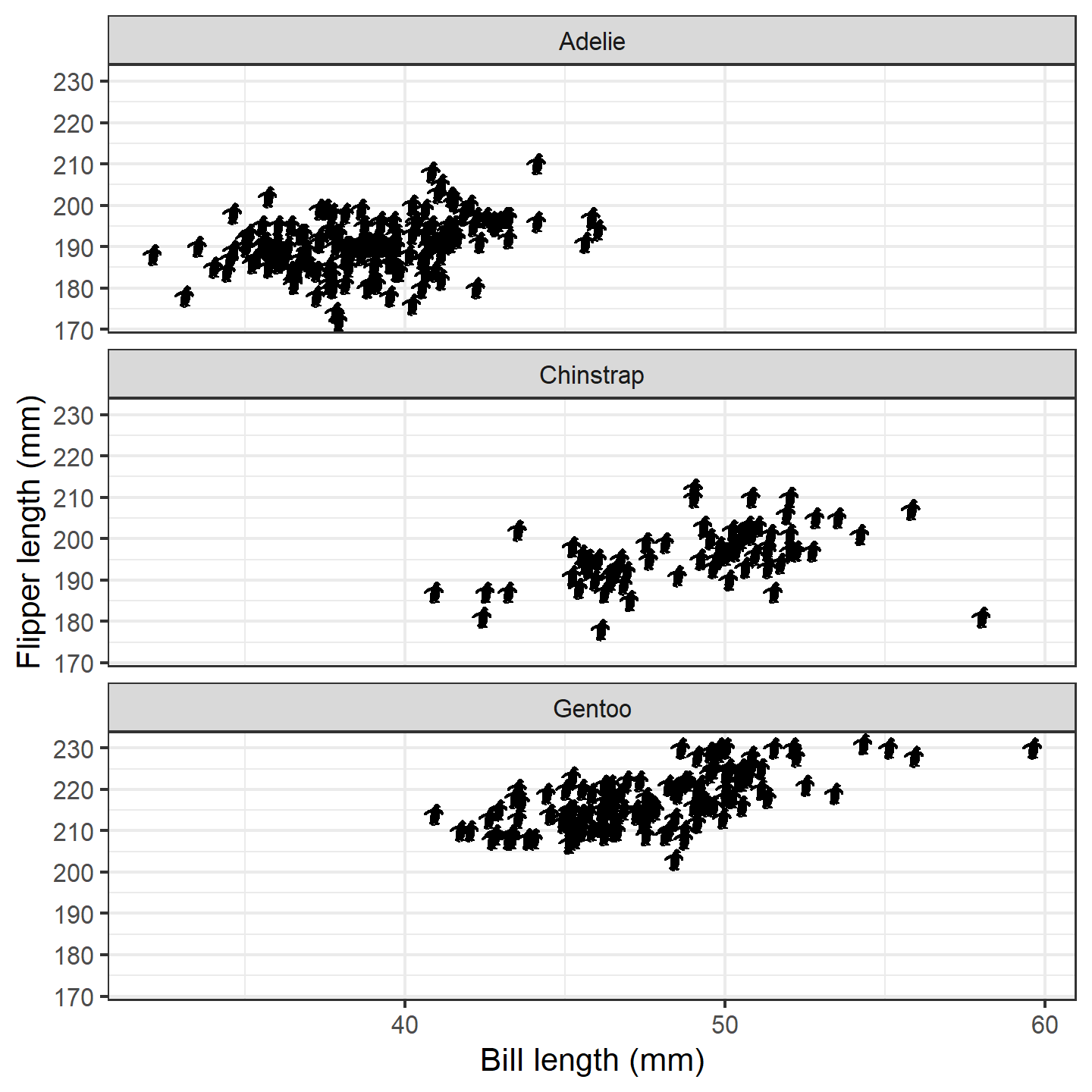plot of chunk ggplot-penguin-plot-3

The default silhouette size for geom_phylopic() is 1.5 which appears to work well given the range of the y-axis here. However, we can also vary the size based on some other aspect of data. In this case, let’s try making the size of the silhouettes relative to the penguins’ body masses. {ggplot2} should work its magic and make them reasonable sizes for the plot:

ggplot(penguins_subset) +
geom_phylopic(img = penguin_rot,
aes(x = bill_length_mm, y = flipper_length_mm,
size = body_mass_g)) +
labs(x = "Bill length (mm)", y = "Flipper length (mm)") +
facet_wrap(~species, ncol = 1) +
theme_bw(base_size = 15)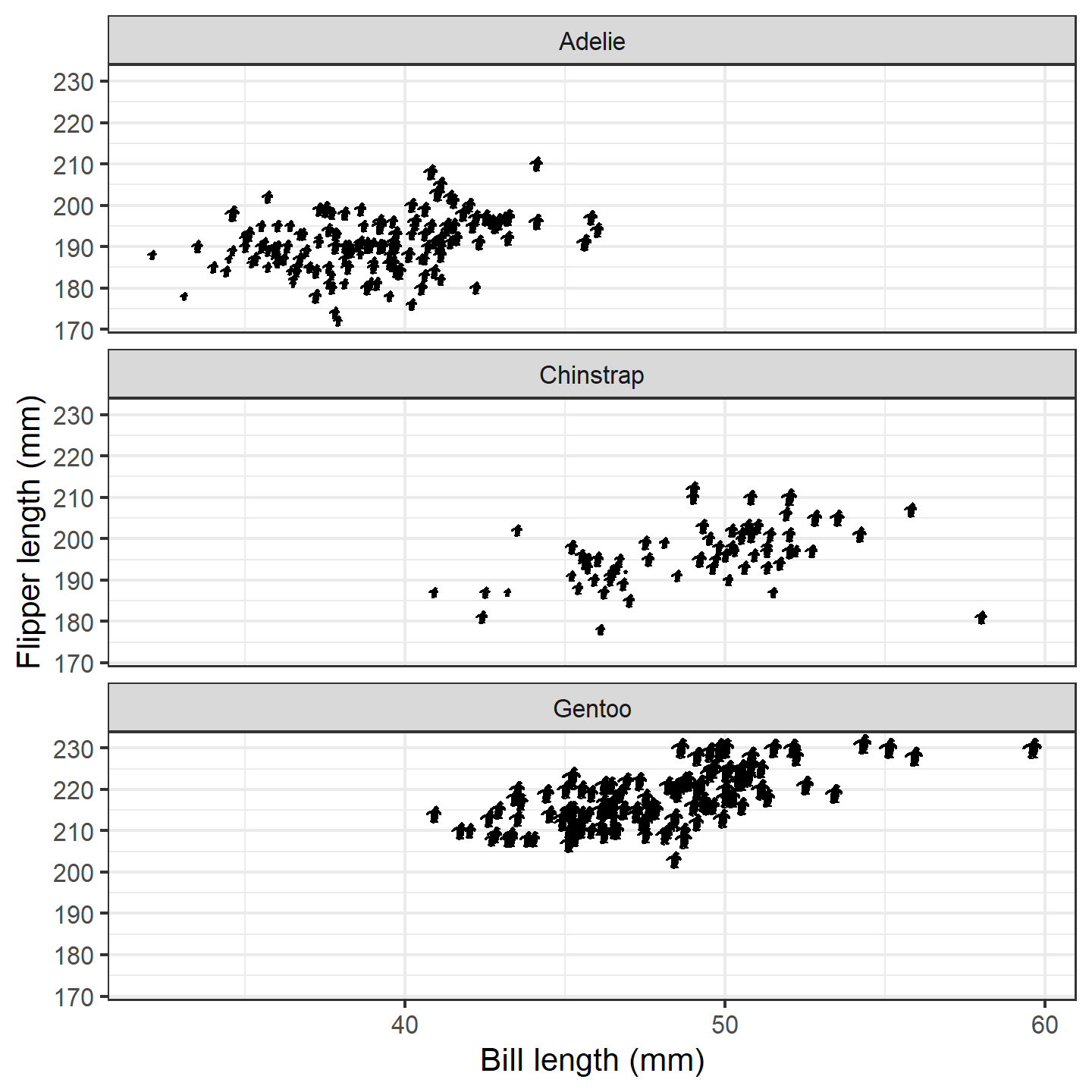plot of chunk ggplot-penguin-plot-4

Finally, let’s color the female and male penguins with different colors. Note that the default for geom_phylopic() is to not display a legend, so we need to set show.legend = TRUE. However, we only want a legend for the colors, so we use guide = "none" for the size scale:

ggplot(penguins_subset) +
geom_phylopic(img = penguin_rot,
aes(x = bill_length_mm, y = flipper_length_mm,
size = body_mass_g, color = sex),
show.legend = TRUE) +
labs(x = "Bill length (mm)", y = "Flipper length (mm)") +
scale_size_continuous(guide = "none") +
scale_color_manual("Sex", values = c("orange", "blue"),
labels = c("Female", "Male")) +
facet_wrap(~species, ncol = 1) +
theme_bw(base_size = 15) +
theme(legend.position = c(0.9, 0.9))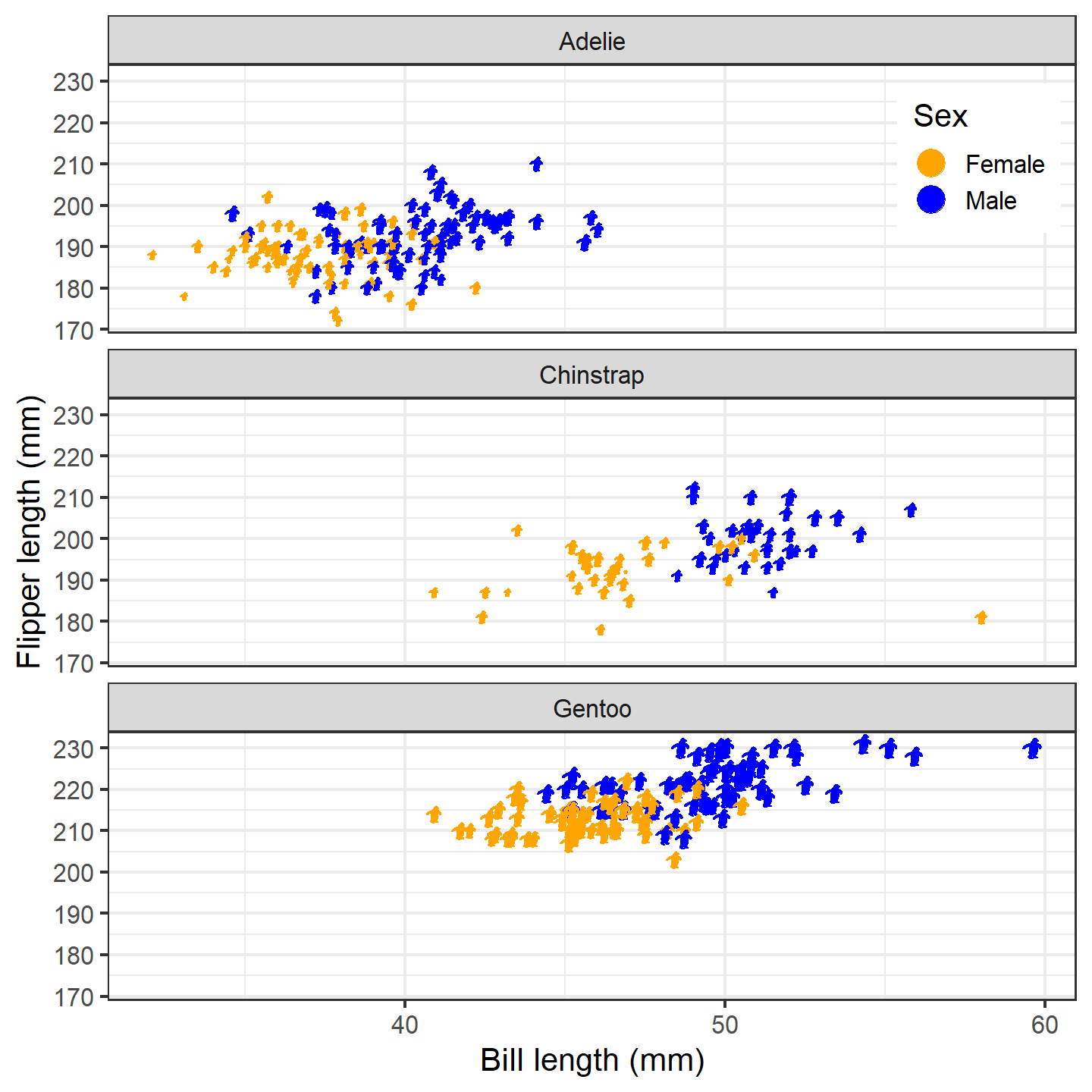plot of chunk ggplot-penguin-plot-5

Hmm…the colored dots in the legend are great, but lucky for us, the package also supplies a convenient way to include silhouettes in the legend. Due to technical constraints, you’ll need to specify the images/uuids/names again within phylopic_key_glyph(). If you supply more than one silhouette to this function, it will cycle through them as it generates legend keys (recycling as needed). Note that phylopic_key_glyph() does not currently support the size aesthetic.

ggplot(penguins_subset) +
geom_phylopic(img = penguin_rot,
aes(x = bill_length_mm, y = flipper_length_mm,
size = body_mass_g, color = sex),
show.legend = TRUE,
key_glyph = phylopic_key_glyph(img = penguin_rot)) +
labs(x = "Bill length (mm)", y = "Flipper length (mm)") +
scale_size_continuous(guide = "none") +
scale_color_manual("Sex", values = c("orange", "blue"),
labels = c("Female", "Male")) +
facet_wrap(~species, ncol = 1) +
theme_bw(base_size = 15) +
theme(legend.position = c(0.9, 0.9))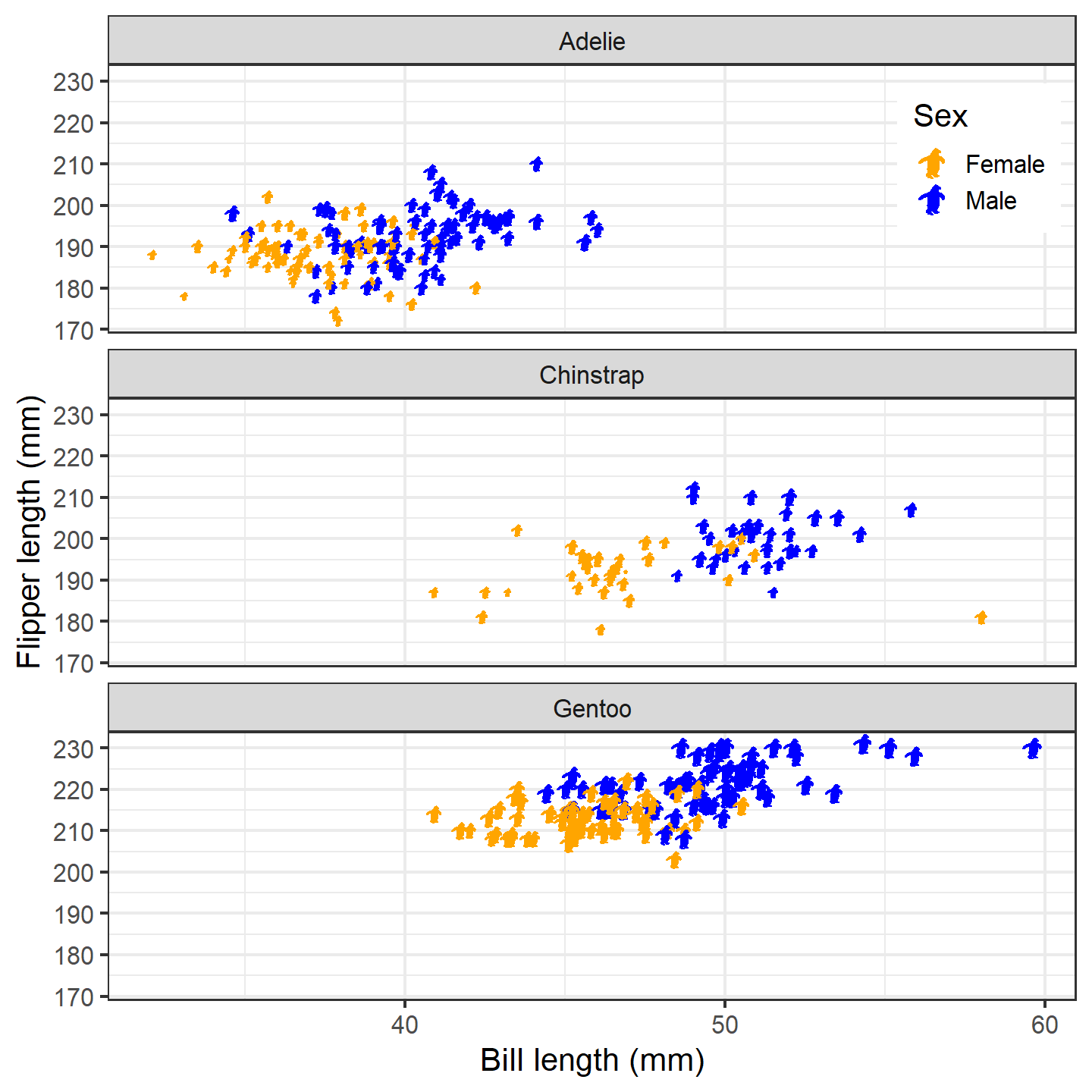plot of chunk ggplot-penguin-plot-6

Now that’s a nice figure!

# Geographic distribution

In much the same way as generic x-y plotting, the rphylopic package can be used in combination with {ggplot2} to plot organism silhouettes on a map. That is, to plot data points (e.g., species occurrences) as silhouettes. We provide an example here of how this might be achieved. For this application, we use the example occurrence dataset of early (Carboniferous to Early Triassic) tetrapods from the {palaeoverse} R package to visualize the geographic distribution of Mesosaurus fossils.

First, let’s load our libraries and the tetrapod data:

# Load libraries
library(rphylopic)
library(ggplot2)
library(palaeoverse)
# Get occurrence data
data(tetrapods)

Then we’ll subset our occurrences to only those for Mesosaurus:

# Subset to desired group
tetrapods <- subset(tetrapods, genus == "Mesosaurus")

Now, let’s plot those occurrences on a world map. {ggplot2} and it’s built-in function map_data() make this a breeze. Note that we use alpha = 0.75 in case there are multiple occurrences in the same place. That way, the darker the color, the more occurrences in that geographic location.

# Get map data
world <- map_data(map = "world")
# Make map
ggplot() +
geom_polygon(data = world, aes(x = long, y = lat, group = group),
fill = "lightgray", color = "darkgrey", linewidth = 0.1) +
geom_point(data = tetrapods, aes(x = lng, y = lat),
size = 4, alpha = 0.75, color = "blue") +
theme_void() +
coord_sf()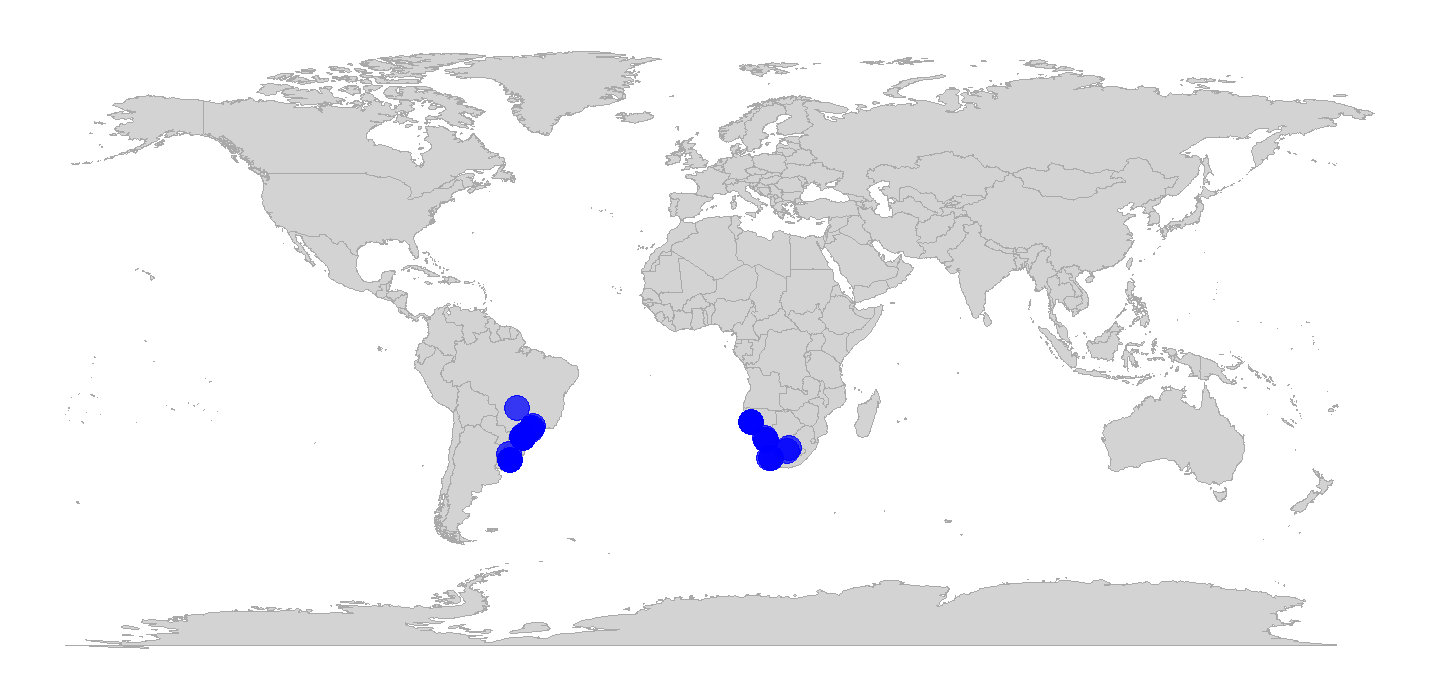plot of chunk ggplot-geog-plot-1

Now, as with the penguin figure above, we can easily replace those points with silhouettes.

ggplot() +
geom_polygon(data = world, aes(x = long, y = lat, group = group),
fill = "lightgray", color = "darkgrey", linewidth = 0.1) +
geom_phylopic(data = tetrapods, aes(x = lng, y = lat, name = genus),
size = 4, alpha = 0.75, color = "blue") +
theme_void() +
coord_sf()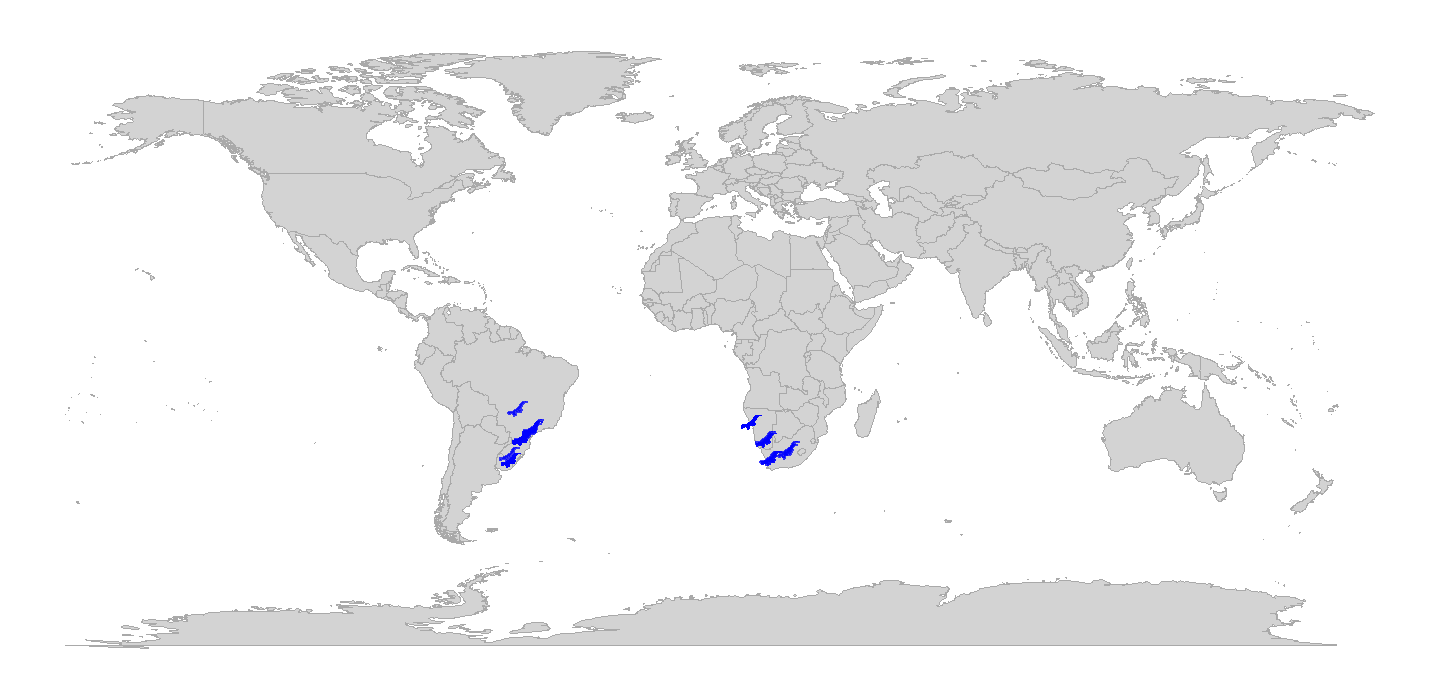plot of chunk ggplot-geog-plot-2

Snazzy!

Note that while we used the genus name as the name aesthetic here, we easily could have done name = "Mesosaurus" outside of the aes() call instead. However, if we were plotting occurrences of multiple genera, we’d definitely want to plot them as different silhouettes using name = genus within the aes() call.

Also, note that we could change the projection of the map and data using the crs and default_crs arguments in coord_sf(). When projecting data, note that the y-axis limits will change to projected limits. For example, in the Robinson projection, the y-axis limits are roughly -8,600,000 and 8,600,000 in projected coordinates. Therefore, you may need to adjust the size argument/aesthetic accordingly when projecting maps and data.

# Phylogenetics

Another common use case of PhyloPic silhouettes is to represent taxonomic information. In this example, we demonstrate how to use silhouettes within a phylogenetic framework. In this case, the phylogeny, taken from the {phytools} package, includes taxa across all vertebrates. Even many taxonomic experts are unlikely to know the scientific names of these 11 disparate taxa, so we’ll replace the names with PhyloPic silhouettes. First, let’s load our libraries and data:

# Load libraries
library(rphylopic)
library(ggplot2)
library(phytools)
# Get vertebrate phylogeny and data
data(vertebrate.tree)

We can use a vectorized version of the get_uuid() function to retrieve UUID values for all of the species at once. However, just in case we get an error, we wrap the get_uuid() call in a tryCatch() call. This way, we should get either a UUID or NA for each species:

# Make a data.frame for the PhyloPic names
vertebrate_data <- data.frame(species = vertebrate.tree$tip.label, uuid = NA) # Try to get PhyloPic UUIDs for the species names vertebrate_data$uuid <- sapply(vertebrate.tree$tip.label, function(x) { tryCatch(get_uuid(x), error = function(e) NA) }) vertebrate_data ## species uuid ## 1 Carcharodon_carcharias 00f208a3-887d-4ae8-838c-2124f53b9fc1 ## 2 Carassius_auratus b1995423-0170-4ff1-af49-1cdf692d8fc7 ## 3 Latimeria_chalumnae 12c38a8a-6d68-4af3-ada3-05cafdfc25c2 ## 4 Homo_sapiens 9c6af553-390c-4bdd-baeb-6992cbc540b1 ## 5 Lemur_catta 8a187391-82a3-4d9b-a402-3a310bf7dc38 ## 6 Myotis_lucifugus <NA> ## 7 Sus_scrofa 3d8acaf6-4355-491e-8e86-4a411b53b98b ## 8 Megaptera_novaeangliae 012afb33-55c3-4fc6-9ae3-3a91fda32fd5 ## 9 Bos_taurus dc5c561e-e030-444d-ba22-3d427b60e58a ## 10 Iguana_iguana 5dec03d9-66a2-4033-b1a9-6dbb3485199f ## 11 Turdus_migratorius 83b29bf0-f4f9-412d-8b3b-7faf4febd69d Oh no, we weren’t able to find a silhouette for Myotis lucifugus (little brown bat)! Good thing we used tryCatch()! Given the coarse resolution of this phylogeny, we can just grab a silhouette for the subfamily (Vespertilioninae): vertebrate_data$uuid[vertebrate_data$species == "Myotis_lucifugus"] <- get_uuid("Vespertilioninae") I’m also not a huge fan of the boar picture. Let’s choose an alternative with pick_phylopic(). # Pick a different boar image; we'll pick #2 boar_svg <- pick_phylopic("Sus scrofa", view = 5) # Extract the UUID vertebrate_data$uuid[vertebrate_data$species == "Sus_scrofa"] <- attr(boar_svg, "uuid") Now that we’ve got our phylogeny and UUIDs, we could go ahead and create our figure. However, time for a quick aside. The time required for geom_phylopic() and the other rphylopic visualization functions scales with the number of unique names/UUIDs, not the number of plotted silhouettes. Therefore, if you are plotting a lot of different silhouettes, these functions can take quite a long time to poll PhyloPic for each unique name, download the silhouettes, and convert them to be added to the plot. If you plan to use the same silhouettes for multiple figures, we strongly suggest that you poll PhyloPic yourself using get_phylopic() or pick_phylopic(), save the silhouettes to your R environment, and then these use image objects in the visualization functions (with the img argument/aesthetic). Following this advice, let’s get image objects for these 11 species before we make our figure. Note that, since we’ve used get_uuid() to get these 11 UUIDs, we know that they are valid, so we don’t need to catch any errors this time. vertebrate_data$svg <- lapply(vertebrate_data$uuid, get_phylopic) Now let’s go ahead and plot our phylogeny with the ggtree package: library(ggtree) # Plot the tree ggtree(vertebrate.tree, size = 1, layout = "circular")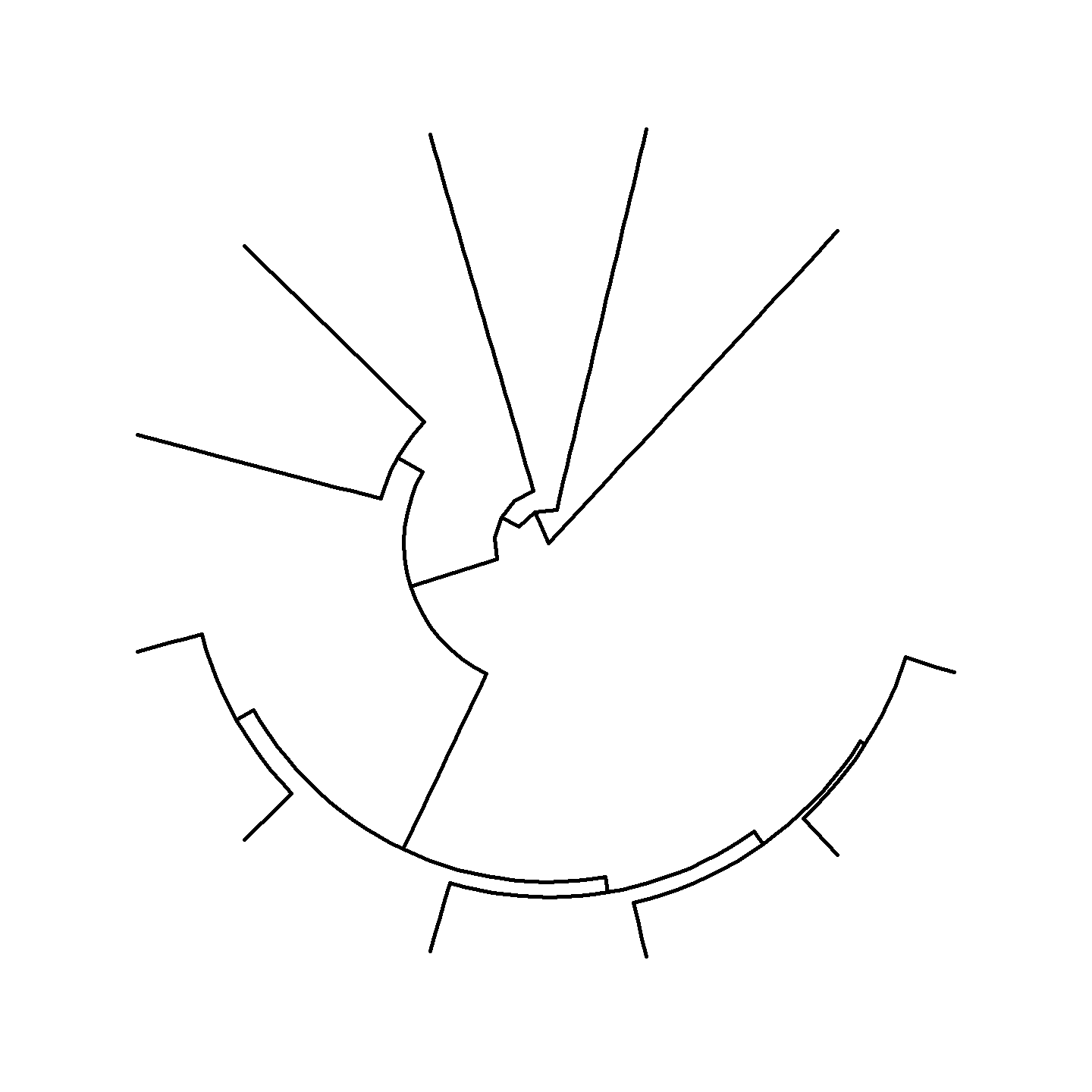plot of chunk ggplot-phylo-plot-1 Hmm…that’s a bit boring. Let’s add a geological timescale to the background using coord_geo_polar() from the {deeptime} package. Note that we need to use the revts() function to reverse the time axis to work with coord_geo_polar(). library(deeptime) # Plot the tree with a geological timescale in the background revts(ggtree(vertebrate.tree, size = 1)) + scale_x_continuous(breaks = seq(-500, 0, 100), labels = seq(500, 0, -100), limits = c(-500, 0)) + coord_geo_polar(dat = "periods") + theme(line = element_line(linewidth = 1), axis.text.r = element_text(size = 5, hjust = -0.5, vjust = -1.5))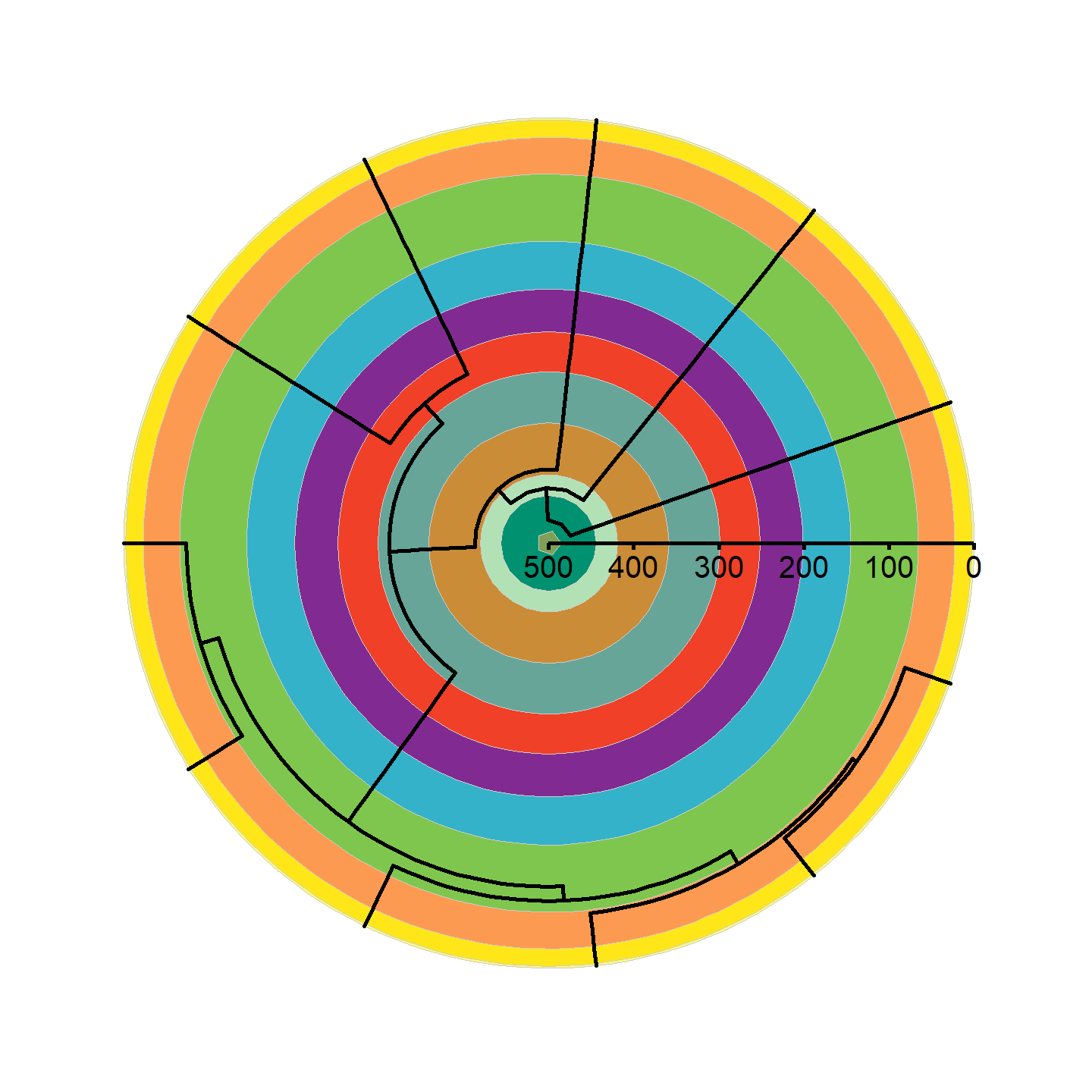plot of chunk ggplot-phylo-plot-2 That’s looking a lot prettier! Let’s go ahead and add our silhouettes now. Note that we need to attach the vertebrate_data object with the %<+% operator from {ggtree}. revts(ggtree(vertebrate.tree, size = 1)) %<+% vertebrate_data + geom_phylopic(aes(img = svg), size = 25) + scale_x_continuous(breaks = seq(-500, 0, 100), labels = seq(500, 0, -100), limits = c(-500, 0)) + coord_geo_polar(dat = "periods") + theme(line = element_line(linewidth = 1), axis.text.r = element_text(size = 5, hjust = -0.5, vjust = -1.5))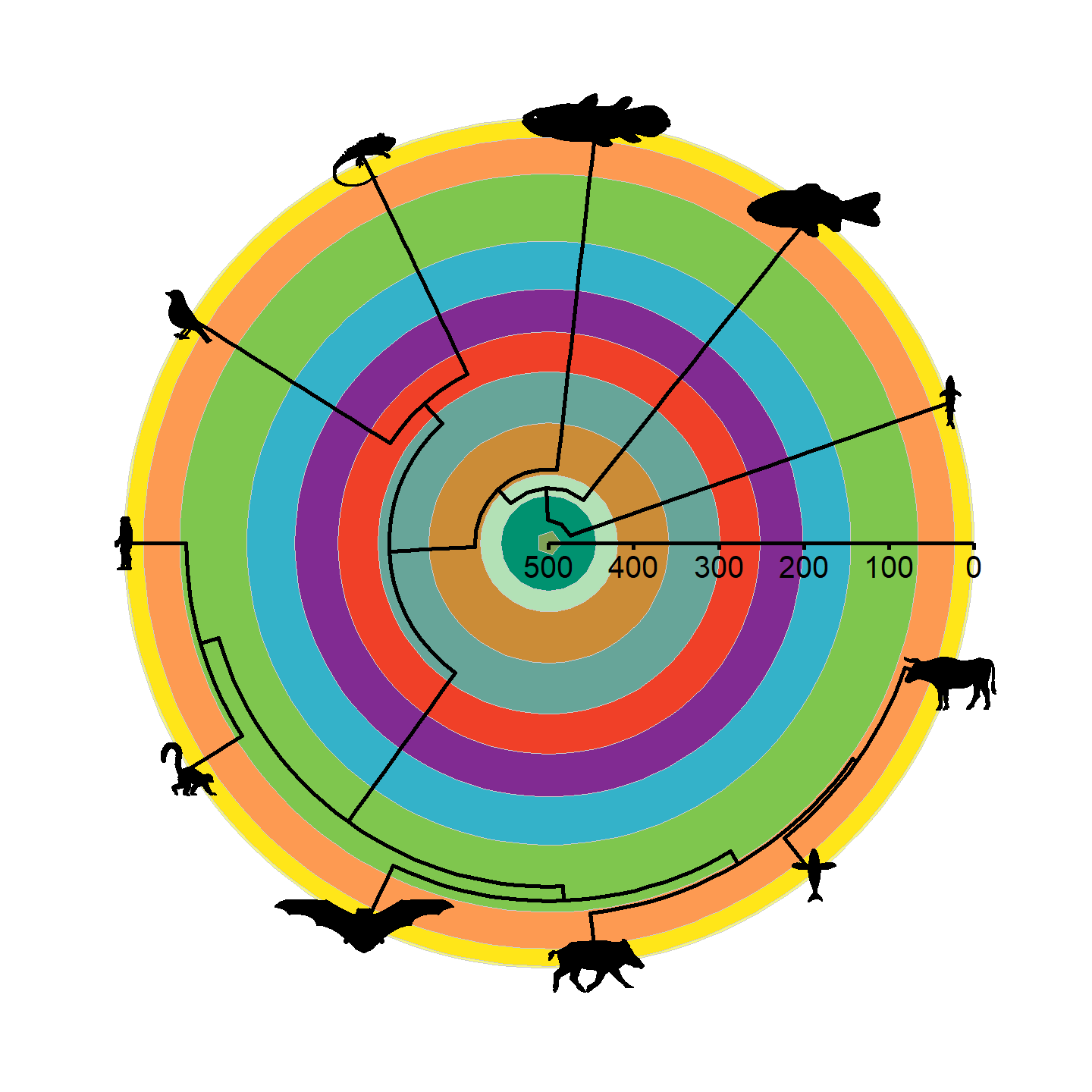plot of chunk ggplot-phylo-plot-3 Note that only a single size is specified and aspect ratio is always maintained, hence why the silhouettes all have the same height but different widths. Let’s fix some of the silhouettes by rotating them 90 degrees: vertebrate_data$svg[] <- rotate_phylopic(img = vertebrate_data$svg[]) vertebrate_data$svg[] <- rotate_phylopic(img = vertebrate_data\$svg[])

And now the finished product:

revts(ggtree(vertebrate.tree, size = 1)) %<+% vertebrate_data +
geom_phylopic(aes(img = svg), size = 25) +
scale_x_continuous(breaks = seq(-500, 0, 100),
labels = seq(500, 0, -100),
limits = c(-500, 0)) +
coord_geo_polar(dat = "periods") +
theme(line = element_line(linewidth = 1),
axis.text.r = element_text(size = 5, hjust = -0.5, vjust = -1.5))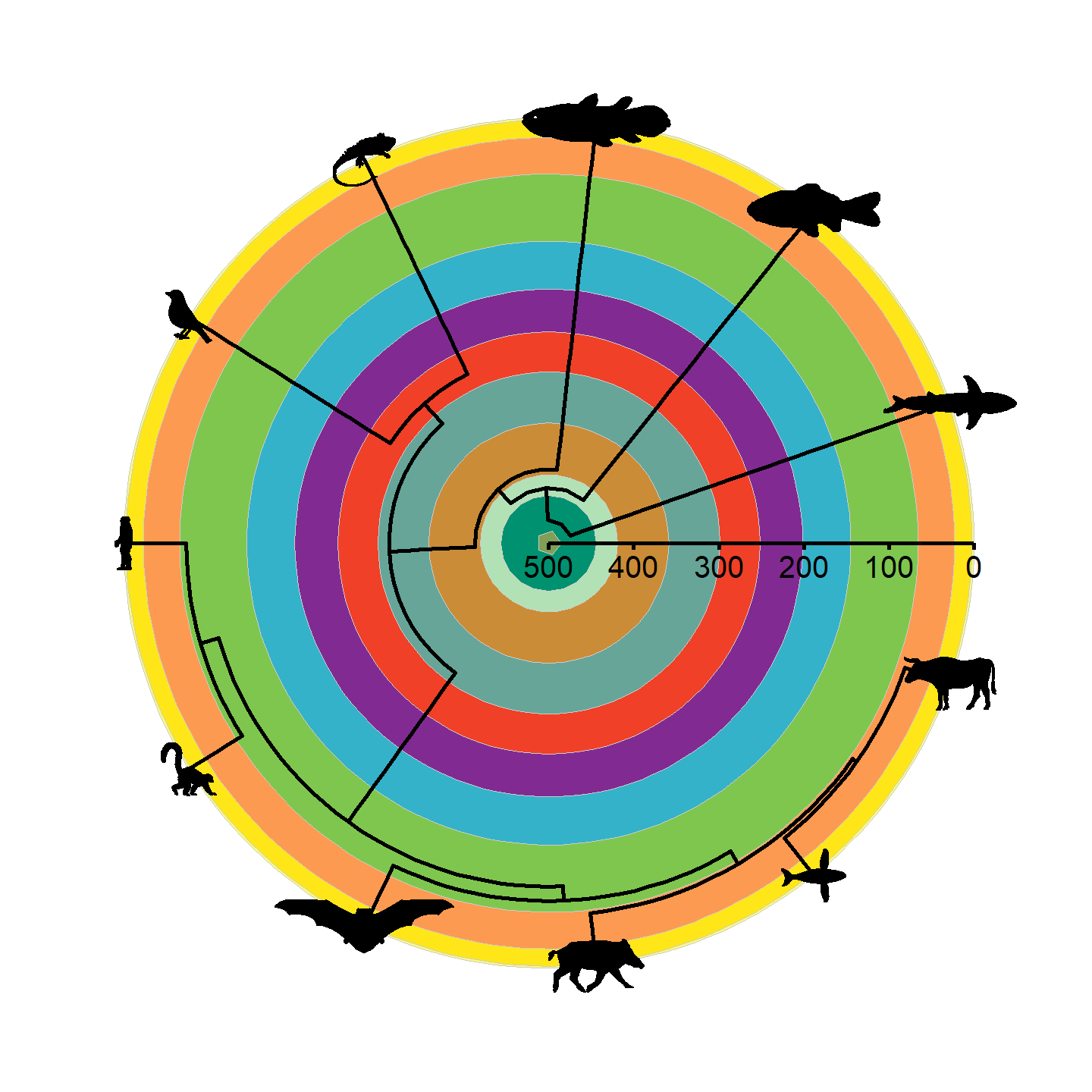plot of chunk ggplot-phylo-plot-4

Beautiful!